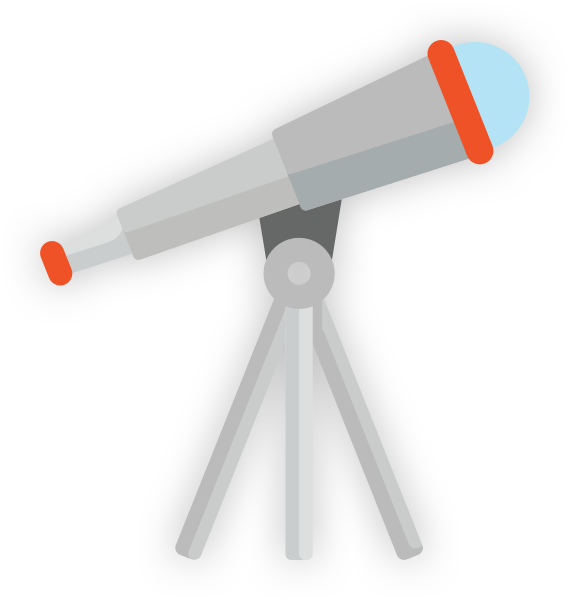# Power

## Definition of Power

When a device is working, it is converting energy to perform its function. The rate at which it converts energy is known as the power of the device.

Power is the rate of energy transfer measured in Watts (W). 1 Watt is 1 Joule per second

All light bulbs do the same overall function, they transfer electrical energy to light and heat energies. Old fashioned light bulbs were classified by their Wattage, to indicate how bright they would be, so a 60W bulb would be less bright than a 100 W bulb. The 60 W converts 60 joules of electrical energy to light and heat a second. A modern LED bulb is more efficient, they achieve the same brightness from a 5 W LED. Thus they only convert 5 joules of energy a seconds, making them much cheaper to use.

Examples

 Device Power rating (Watts) TV 150 TV on standby 1 PC with hard disk 100 PC with solid state HDD 75 Fan Heater 3000 Kettle 1200- 3000 Dishwasher 1200 - 1500 Games console 120 200 Microwave cooking 600-1700 Microwave on standby 3 Phone charger 4 -7 Fridge 100 - 200

Any device that involves generating heat have high power ratings. Devices on standby are still converting electrical energy and so have a power rating.

Any device or system that is transferring energy has a power rating, not just electrical devices. A steam train is converting chemical energy via heat to kinetic energy, originally the idea of power was applied to steam engines. James Watt whose name is used for the unit of power was an engineer working on steam engines.The image is a Watt Steam Engine.

## Calculating Power

Power is the rate of energy transfer and can be calculated using the following formula.

_Power = Energy transferred ÷ time taken P = E ÷ t measured in Watts (W). _

Example 1:

A TV transfers 2.3 MJ of electricity into heat, light and sound in an evening over a period of 4 hours. What is the power rating of the TV?

All figures must in SI units. Time’s SI unit is the second, so before using the formula the 4 hours must be changed to seconds ( 4 hours x 3,600 seconds per hour = 14,400 s)

P = E ÷ t _ = 2,300,000 _J ÷ 14,400 s = 159.72 W

Example 2:

A student spends 2 hours on a game console connected to a TV. At the same time the laptop is on for him to do his revision. Using the data in the table, calculate the total energy transferred in this 2 hour period by these devices.

 Device Power rating (Watts) TV 150 TV on standby 1 PC with solid state HDD 75 Games console 200

Time in seconds = 2 hours x 3,600 seconds per hour = 7,200 s

Power of devices TV(150) + Console (200) + Laptop (75) = 425 W

P = E ÷ t so E = Pt _= 425 x 7,200 = 3,060,000 _J

## Efficiency

When energy is transferred by a device or system the proportion of the output energy that is able to perform a useful task compared to the energy input to the system is the efficiency of the system.

The more efficient a system or device is the more useful energy is produced and there is less waste energy. An old fashioned filament light bulb is able to produce around 10 J of light for every 100 J of electricity, an LED bulb can produce 95 J of light from 100 J of electricity. The LED is much more efficient. This very low efficiency of filament bulbs is the main reason they are banned from sale in the UK and the EU.There are no units for efficiency, the figure is either presented as a decimal or a percentage.In the exam if you calculate an efficiency with a value greater than 1 or 100% you have made an mistake. No system can be more than 100 % efficient. Check your calculations you might have the numbers the wrong way round.

Example 1:

A laptop converts 6,000 J of electricity over an hour, of which 2,000 J are converted to heat, the rest is converted to light from the screen, sounds from the speakers and electromagnetic energy for the wi-fi. How efficient is the laptop?

The only waste energy is the heat so from the 6,000 J, 4,000 J are converted to useful forms of energy.

Efficiency = Useful energy ÷ Total energy = 4,000 / 6,000 = 0.67 or 67 %

Example 2:

A kettle has an efficiency rating of 0.85, it transfers a total of 252 KJ of electrical energy in 3 minutes to boil the water. How much of the energy has be converted to useful heat energy?Efficiency = Useful energy ÷ Total energy

so Useful Energy = Efficiency x Total energy

Useful Energy (Heat) = 0.85 x 252, 000 J = 214,200 J

Define the terms; power and efficiency.